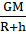Fill in the blanks and explain the statements with reasoning: a. If the height of the orbit of a satellite from the earth surface is increased, the tangential velocity of the satellite will …

Question 1.

Fill in the blanks and explain the statements with reasoning:

a. If the height of the orbit of a satellite from the earth surface is increased, the tangential velocity of the satellite will …

b. The initial velocity (during launching) of the Mangalyaan, must be greater than …………..of the earth.

(a) If the height of the orbit of a satellite from the earth surface is increased, the tangential velocity of the satellite will decreases.

Explanation: As tangential velocity of satellite is inversely proportional to the height of orbit of satellite from earth’s surface.

V= √Where V = tangential velocity

G = gravitational constant

M = mass of earth